# AP Board 7th Class Maths Solutions Chapter 7 Data Handling Ex 4

AP State Syllabus AP Board 7th Class Maths Solutions Chapter 7 Data Handling Ex 4 Textbook Questions and Answers.

## AP State Syllabus 7th Class Maths Solutions 7th Lesson Data Handling Exercise 4Question 1.
Draw a bar graph for the following data.
Population of India in successive census years-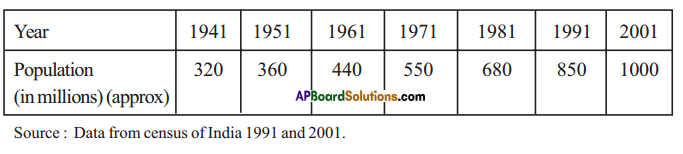Solution: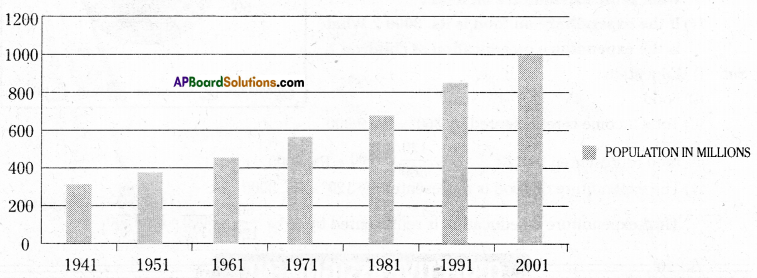Question 2.
Draw a pie chart for the following data.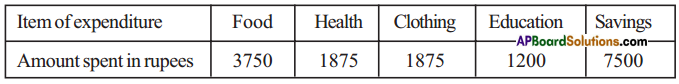Solution: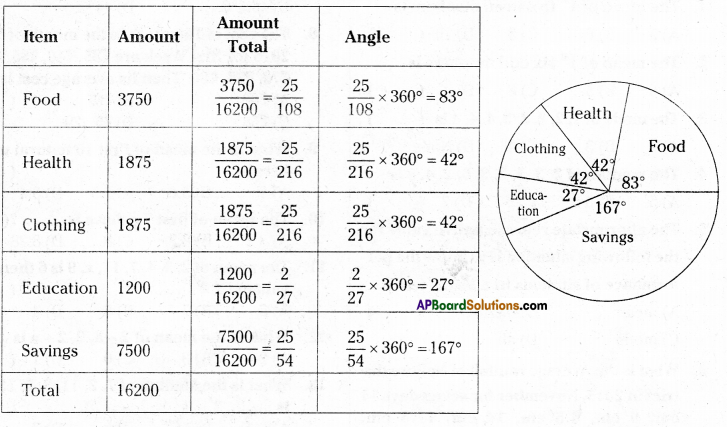Question 3.
Draw a double bar graph for the following data.
Birth and Death rates of different states in 1999.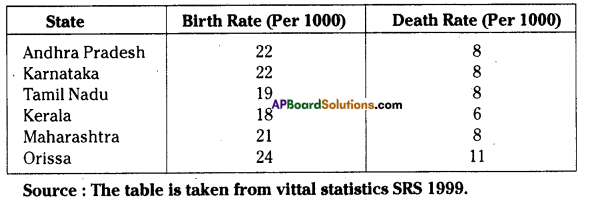Solution: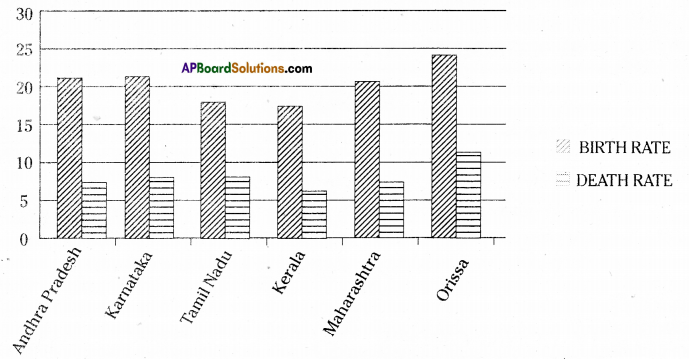Question 4.
Draw a pie chart for the following data.
Time spent by a child during a day-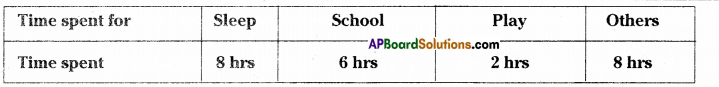Solution: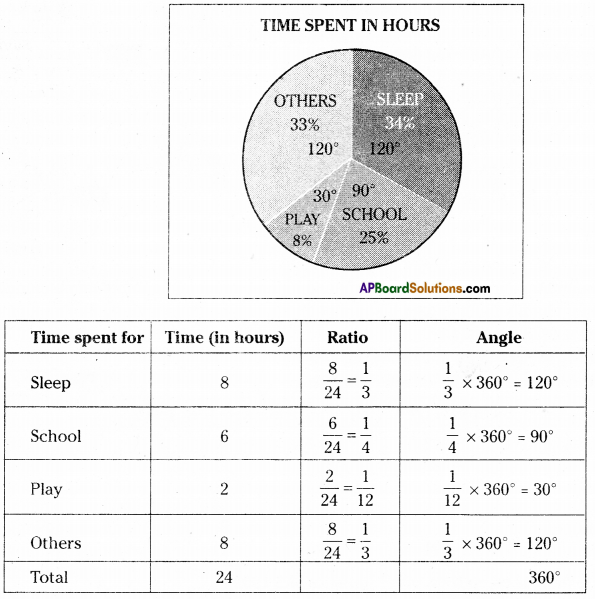Question 5.
The adjoining pic chart gives the expenditure on various items during a month for a family.
(The numbers written around the pie chart tell us the angles made by each sector at the centre.)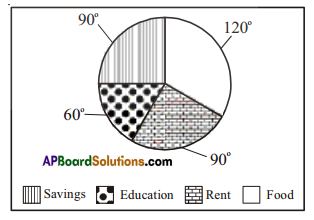food represented by 120 = $$\frac{120}{360}$$ × 9000 = Rs. 3000
Then expenditure on education is represented by $$\frac{60}{120}$$ × 3000 = Rs. 1500